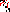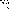MINLPLib

A Library of Mixed-Integer and Continuous Nonlinear Programming Instances

Instance: alkylation

 Formatsⓘ ams gms mod nl osil Primal Bounds (infeas ≤ 1e-08)ⓘ 1768.80696400 p1 ( gdx sol ) (infeas: 7e-15) Other points (infeas > 1e-08)ⓘ Dual Boundsⓘ 1768.80696500 (ANTIGONE)1768.80701500 (BARON)1768.80699200 (COUENNE)1768.80852000 (LINDO)1780.09464700 (SCIP) Referencesⓘ Edgar, T F, Himmelblau, D M, and Lasdon, L S, Optimization of Chemical Processes, McGraw Hill, Boston, 2001. Sourceⓘ Housam Binous Added to libraryⓘ 31 Jul 2001 Problem typeⓘ NLP #Variablesⓘ 10 #Binary Variablesⓘ 0 #Integer Variablesⓘ 0 #Nonlinear Variablesⓘ 8 #Nonlinear Binary Variablesⓘ 0 #Nonlinear Integer Variablesⓘ 0 Objective Senseⓘ max Objective typeⓘ quadratic Objective curvatureⓘ indefinite #Nonzeros in Objectiveⓘ 6 #Nonlinear Nonzeros in Objectiveⓘ 2 #Constraintsⓘ 11 #Linear Constraintsⓘ 5 #Quadratic Constraintsⓘ 2 #Polynomial Constraintsⓘ 2 #Signomial Constraintsⓘ 0 #General Nonlinear Constraintsⓘ 2 Operands in Gen. Nonlin. Functionsⓘ mul div Constraints curvatureⓘ indefinite #Nonzeros in Jacobianⓘ 31 #Nonlinear Nonzeros in Jacobianⓘ 12 #Nonzeros in (Upper-Left) Hessian of Lagrangianⓘ 19 #Nonzeros in Diagonal of Hessian of Lagrangianⓘ 5 #Blocks in Hessian of Lagrangianⓘ 2 Minimal blocksize in Hessian of Lagrangianⓘ 4 Maximal blocksize in Hessian of Lagrangianⓘ 4 Average blocksize in Hessian of Lagrangianⓘ 4.0 #Semicontinuitiesⓘ 0 #Nonlinear Semicontinuitiesⓘ 0 #SOS type 1ⓘ 0 #SOS type 2ⓘ 0 Infeasibility of initial pointⓘ 0.44 Sparsity JacobianⓘSparsity Hessian of Lagrangianⓘ\$offlisting
*
*  Equation counts
*      Total        E        G        L        N        X        C        B
*         12        4        8        0        0        0        0        0
*
*  Variable counts
*                   x        b        i      s1s      s2s       sc       si
*      Total     cont   binary  integer     sos1     sos2    scont     sint
*         11       11        0        0        0        0        0        0
*  FX      0
*
*  Nonzero counts
*      Total    const       NL      DLL
*         38       24       14        0
*
*  Solve m using NLP maximizing objvar;

Variables  x1,x2,x3,x4,x5,x6,x7,x8,x9,x10,objvar;

Equations  e1,e2,e3,e4,e5,e6,e7,e8,e9,e10,e11,e12;

e1.. -0.063*x4*x7 + 5.04*x1 + 0.035*x2 + 10*x3 + 3.36*x5 + objvar =E= 0;

e2..    x1 - 1.22*x4 + x5 =E= 0;

e3.. -98000*x3/(x4*x9 + 1000*x3) + x6 =E= 0;

e4.. -(x2 + x5)/x1 + x8 =E= 0;

e5.. (1.12 + 0.13167*x8 - 0.00667*x8**2)*x1 - 0.99*x4 =G= 0;

e6.. -(1.12 + 0.13167*x8 - 0.00667*x8**2)*x1 + 1.01010101010101*x4 =G= 0;

e7.. 1.098*x8 - 0.038*x8**2 + 0.325*x6 - 0.99*x7 =G= -57.425;

e8.. -(1.098*x8 - 0.038*x8**2) - 0.325*x6 + 1.01010101010101*x7 =G= 57.425;

e9..  - 0.9*x9 - 0.222*x10 =G= -35.82;

e10..    1.11111111111111*x9 + 0.222*x10 =G= 35.82;

e11..    3*x7 - 0.99*x10 =G= 133;

e12..  - 3*x7 + 1.01010101010101*x10 =G= -133;

* set non-default bounds
x1.lo = 1E-6; x1.up = 2000;
x2.lo = 1E-6; x2.up = 16000;
x3.lo = 1E-6; x3.up = 120;
x4.lo = 1E-6; x4.up = 5000;
x5.lo = 1E-6; x5.up = 2000;
x6.lo = 85; x6.up = 93;
x7.lo = 90; x7.up = 95;
x8.lo = 3; x8.up = 12;
x9.lo = 0.01; x9.up = 4;
x10.lo = 145; x10.up = 162;

* set non-default levels
x1.l = 1745;
x2.l = 12000;
x3.l = 110;
x4.l = 3048;
x5.l = 1974;
x6.l = 89.2;
x7.l = 92.8;
x8.l = 8;
x9.l = 3.6;

Model m / all /;

m.limrow=0; m.limcol=0;
m.tolproj=0.0;

\$if NOT '%gams.u1%' == '' \$include '%gams.u1%'

\$if not set NLP \$set NLP NLP
Solve m using %NLP% maximizing objvar;

Last updated: 2021-11-30 Git hash: 8337812f